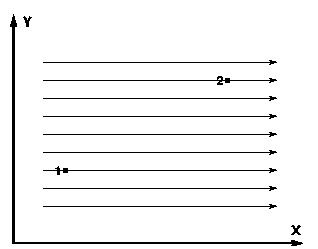# Problem: The figure below shows two points in an E-field:Point 1 is at (X1, Y1) = (3,4) in m, andPoint 2 is at (X2, Y2) = (12,9) in m. The Electric Field is constant, with a magnitude of 77 V/m, and is directed parallel to the +x-axis. The potential at point 1 is 1200 V.1. Calculate the potential at point 2.2. Calculate the work required to move a negative charge of Q = -585 μC from point 1 to point 2.

###### FREE Expert Solution

In this problem, we are dealing with electric field, change in potential, and work.

Work:

100% (216 ratings)###### Problem Details

The figure below shows two points in an E-field:

Point 1 is at (X1, Y1) = (3,4) in m, and

Point 2 is at (X2, Y2) = (12,9) in m.

The Electric Field is constant, with a magnitude of 77 V/m, and is directed parallel to the +x-axis. The potential at point 1 is 1200 V.

1. Calculate the potential at point 2.

2. Calculate the work required to move a negative charge of Q = -585 μC from point 1 to point 2.